# IST Linear Algebra - Speakers and Syllabus

This school is mainly addressed to lecturers and young researchers. Linear Algebra is a beautiful and basic part of mathematics. Notions and techniques of linear algebra have wide applicability both within and outside mathematics.  It is our intention to give a fairly comprehensive introduction to Linear Algebra in two weeks. We plan to start from scratch, but progress rapidly to give a substantive introduction of fundamental topics in linear algebra, and provide glimpses of multilinear algebra as well as some of the applications of linear algebra. This school will provide an opportunity to university lecturers and young researchers to consolidate their knowledge and gain a perspective in linear algebra, which could be useful for their teaching and research. The details of the topics to be covered in this school are given below.

Faculty

 Name Affiliation Sarang S. Sane IIT Madras Anathnarayan Hariharan IIT Bombay Sudhir R. Ghorpade IIT Bombay Vikas Bist Panjab University Krishna Kaipa IISER Pune S. A. Katre University of Pune Arunkumar R. Patil SGGS, Nanded Tutors/Associate Teachers Name Affiliation Fernando Pinero IIT Bombay Mrinmoy Datta IIT Bombay Prasant Singh IIT Bombay Vijay Singh IIT Bombay

I. Linear equations and Matrices (Prof.  Sarang  S. Sane)

• Systems of homogeneous and nonhomogeneous linear equations.
• Matrices. Matrix representation of systems oflinear equations.
• Addition, scalar multiplication and multiplication of matrices. Transpose of a matrix.
• Types of matrices: identity matrix, scalar matrices, diagonal matrices, (upper and lower)
• triangular matrices, symmetric matrices and skew-symmetric matrices.
• Definition of invertible (nonsingular) matrices and of the inverse of a square matrix.
• Block multiplication of matrices.
• Elementary row and column operations. Row reduction (of a matrix to its row echelon form). Gaussian elimination.Applications to solving systems of linear equations.
• Elementary matrices. Relation of elementary row operations with elementary matrices. Invertibility of elementary matrices. Examples of the computation of the inverse of a matrix using Gaussian elimination.
• Notions of row rank and column rank.Equivalence of the row rank and the column rank.Invariance of rank upon elementary row or column operations. Examples of computing the rank as well as bases for row spaces/column spaces of a matrix using row reduction.Equivalence of the rank of a matrix and the rank of the associated linear transformation.
• Relation between the solutions of a system of nonhomogeneous linear equations and the associated system of homogeneous linear equations. Necessary and sufficient condition for a system of nonhomogeneous linear equations to have a solution [viz., the rank of the coefficient matrix equals the rank of the augmented matrix].

Equivalence of statements (in which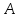denotes an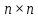matrix) such as the following.

• The system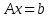of nonhomogeneous linear equations has a unique solution.

• The systemof homogeneous linear equations has no nontrivial solution.

•is invertible

•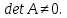•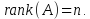Cramer’s Rule. Examples.

II. Vector Spaces and Linear Transformations (Prof. Ananthnarayan Hariharan):

• Review of vectors in the Euclidean plane and 3-space.  Vectors in Rn as  -tuples of real numbers. Addition and scalar multiplication of vectors; basic properties. Length (or the norm) of a vector, unit vector. Dot product, Cauchy-Schwarz inequality. Angle between two vectors. Orthogonal vectors. Projection of a vector onto another vector. Triangle inequality. Pythagoras Theorem. Parallelogram Law. Lines, planes and hyperplanes in Rn .
• Abstract vector spaces over a field. Subspaces; Examples of vector spaces, including the Euclidean space Rn , lines, planes and hyperplanes in Rn passing through the origin, space of solutions of a system of homogeneous linear equations, space of polynomials, spaces of various types of matrices, space of real valued functions on a set. Intersections and sums of subspaces. Direct sums of vector spaces. Quotient space of a vector space by its subspace.
• Linear combination of vectors. Linear span of a subset of a vector space. Definition of a finitely generated vector space. Linear dependence and independence of subsets of a vector space. Basis of a vector space. Basic result that any two bases of a finitely generated vector space have the same number of elements. Dimension of a vector space. Examples. Bases of a vector space as maximal linearly independent sets and as minimal generating sets.
Remarks on infinite dimensional vector spaces and their bases.
• Definition of a linear transformation (homomorphism) of vector spaces; elementary properties. Examples, including linear transformations associated to a rectangular matrix, rotations, reflections and orthogonal projections. Sums and scalar multiples of linear transformations. Composites of linear transformations. Matrix of a linear transformation of finite dimensional vector spaces. Matrix of the composite of two linear transformations. Effect of change of bases. Invertible linear transformations (isomorphisms). Null-space (kernel) and the image (range) of a linear transformation. Nullity and rank of a linear transformation. Rank-Nullity Theorem (Fundamental Theorem of Homomorphisms).
• Linear functionals. Dual bases. Double duals and reflexivity. Adjoint/transpose of a linear transformation.
• Inner product spaces (over R and C  ). Examples. Norm associated to an inner product. Cauchy-Schwarz inequality. Angle between two vectors. Orthogonal sets in an inner product space and their linear independence. Orthonormal bases and the Gram-Schmidt process. Orthogonal complements of subspaces of a finite dimensional inner product space.Orthogonal transformations and orthogonal matrices. Examples.

III. Determinants and Characteristic Polynomials (Prof. Sudhir R. Ghorpade):

• Definition of a determinant of a square matrix as a multilinear, alternating scalar valued function of the rows taking the value 1 at the identity matrix. Uniqueness of such a function. Existence of such a function by defining the determinant via permutations. Geometric interpretation of the determinant as area or volume. Examples.
• Basic properties such as (i) det A=det A,  (ii) det (AB) = (det A) (det B).Laplace expansion of determinants. Special determinants such as van der Monde determinants and circulants.
•  Minors and cofactors. Adjoint of a matrix. The relation  A adj(A) = (detA)I , for a square matrix A and its consequences. Cauchy-Binet formula.
• Eigenvalues and eigenvectors of a linear transformation of a vector space into itself and of square matrices. Characteristic polynomial. Eigenspaces. Algebraic and geometric multiplicity of an eigenvalue. Examples. Similarity of matrices and invariance of characteristic polynomial.
• Cayley-Hamilton Theorem. Minimal polynomial of a matrix. Basic properties. Companion matrices. The notion of diagonalizability. Examples. Triangulation of matrices.

Week 2: November 30 - December 5, 2015

IV. Equivalence, Similarity and Congruence (Prof. Vikas Bist):

• Equivalence of rectangular matrices. Characterization of equivalence classes in terms of rank.
• Similarity classes of square matrices. Characterizations of diagonalizability of a square matrix.
• Projections.
• Invariant subspaces. Primary decomposition theorem.  Annihilators and cyclic subspaces.
• Rational canonical form. Jordan canonical form.
• Orthogonal diagonalizability. Spectral theorem for self-adjoint and normal matrices/operators.
• Congruence of symmetric matrices and quadratic forms. Congruence classes of complex symmetric matrices and of real symmetric matrices. Rank and signature of (real) quadratic forms. Sylvester’s law of inertia.

V. Multilinear Algebra (Prof. Krishna V. Kaipa):

• Symmetric bilinear forms, and associated quadratic forms. Classification over R and C.
• Positive definiteness and positive semidefiniteness. Minorant criteria.
• Alternating bilinear forms and skew-symmetric matrices. Classification over R and C. Pfaffians.
• Multilinear forms. Tensor products of vector spaces. Kronecker product of matrices.
• Symmetric powers and exterior powers. Bases and dimension. Duality.
• Isotropic subspaces, Theorems of Witt.

VI. Applications of Linear Algebra (Dr. Arunkumar R. Patil and Prof. S. A. Katre):

• Vector spaces over finite fields and Linear error correcting codes
• Classification of conic sections and quadric surfaces
• Isometries (rigid motions) of the plane and 3-space.
• Systems of first order linear differential equations
• Perron-Frobenius Theorem and Google Page Rank Algorithm
• Least Squares problems, QR Factorization, Singular Value Decomposition.

TEXTS/REFERENCES

• M. Artin, Algebra, Prentice-Hall of India, 1994.
• S. H. Friedberg, A. J. Insel, and L. E. Spence, Linear Algebra, 4th Ed., Prentice-Hall, 2002.
• P. R. Halmos, Finite Dimensional Vector Spaces, Princeton Univ. Press, 1942.
• F. R. Gantmacher, The Theory of Matrices, Vol. 1 and 2, Chelsea Publishing Co., 1959.
• K. M. Hoffman and R. Kunze, Linear Algebra, 2nd Ed., Prentice-Hall, 1971.
• S. Lang, Linear Algebra, 3rd Ed., Springer-Verlag, 1987.
• P. D. Lax, Linear Algebra and its Applications, 2nd Ed., Wiley-Interscience, 2007.
• S. Roman, Advanced Linear Algebra, 3rd Ed., Springer, 2008.
• G. Strang, Linear Algebra and its Applications, 2nd Ed., Academic Press, 1980.

Time Table for Week 1

 Time Nov 23 Monday Nov 24 Tuesday Nov 25 Wednesday Nov 26 Thursday Nov 27 Friday Nov 28 Saturday 9:00-‐10:00 SSS SSS SSS SSS SSS SSS 10.15-‐11.45 AH AH AH AH AH AH 11:45-­‐12:15 Tea/Coffee 12:15-­‐1:45 SRG SRG SRG SRG SRG SRG Lunch 3:00-­‐5:00 Tutorial (TBA+Tutors) Tutorial (AH+Tutors) Tutorial (SRG+Tutors) Tutorial (TBA+Tutors) Tutorial (AH+Tutors) Tutorial (SRG+Tutors) 5.00-­‐5.30 Tea/Coffee/Snacks

Time Table for Week 2

 Time Nov 30 Monday Dec 1 Tuesday Dec 2 Wednesday Dec 2 Thursday Dec 4Friday Dec 5 Saturday 9:00-‐10:30 VB VB VB VB VB VB 10.30-‐11.00 Tea/Coffee 11:00-­‐12:30 KVK KVK KVK KVK KVK KVK Lunch 2:00-­‐3:00 ARP SAK SAK SAK SAK SAK 3.00-­‐3.30 Tea/Coffee/Snacks 3.30-­‐5.30 Tutorial (VB+Tutors) Tutorial (KVK+Tutors) Tutorial (SAK+Tutors) Tutorial (VB+Tutors) Tutorial (KVK+Tutors) Tutorial (SAK+Tutors)

 Speakers (Week 1) Speakers (Week 2) Tutors SSS Sarang S. Sane VB Vikas Bist Mrinmoy Datta AH Ananthnarayan KVK Kishna V. Kaipa Prasant Singh Hariharan ARP Arunkumar R. Patil Fernando Pinero SRG Sudhir R. Ghorpade SAK S. A. Katre Vijay Singh